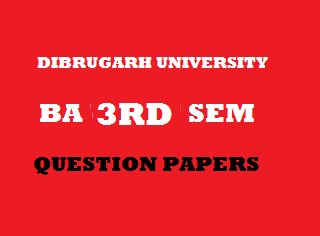## Thursday, January 03, 20192014
(November)
ECONOMICS
(Major)
Course: 301
(Microeconomics - II)
Full Marks: 80
Pass Marks: 32
Time: 3 hours
The figures in the margin indicate full marks for the questions

1. Choose the correct/most appropriate answer of the following: 1x8=8
1. The break-even point for a perfectly competitive firm occurs at the minimum point of the
1. AVC curve.
2. AC curve.
3. MC curve.
4. AFC curve.
1. If an individual seller in a perfectly competitive market wishes to double his sales, he would.
1. Improve the quality of his product.
2. Lower his price to half.
3. Simply offer double the quantity for sale.
4. Advertise the superiority of his product.
1. A queue of a large number of farmers before a single cold storage in the area in a case of
1. Monopoly.
2. Oligopoly.
3. Monopsony.
4. Monopolistic competition.
1. In which one of the following market situations, are the firms mutually interdependent in pricing output decisions?
1. Perfect competition.
2. Monopolistic competition.
3. Monopsony.
4. Oligopoly.
1. Quasi-rent is
1. Equal to the total profits of the firm.
2. Less than the total profits of the firm.
3. The excess of total revenue over total variable cost.
4. The excess of total revenue over total cost.
1. With which one of the following is the doctrine of excess capacity not associated?
1. Perfect competition.
2. Monopolistic competition.
3. Monopoly.
4. Oligopoly.
1. Market failure occurs when there is a divergence between
1. Private costs and social costs.
2. Private gains and social gains.
3. Both (i) and (ii)
4. Neither (i) nor (ii)
1. In the liquidity trap, the interest elasticity of demand for money is
1. Zero.
2. Unity.
3. Infinity.
4. None of the above.
2. Write short notes on any four of the following (within 150 words each): 4x4=16
1. Objectives of the firm.
2. Bilateral monopoly.
3. Monopolistic competition and economic efficiency.
4. Collective bargaining.
5. General equilibrium.
Answer the following questions (within 500 words each):
3. (a) Graphically explain ‘break-even point’ and ‘shutdown point’ of a competitive firm. 6+6=12
Or
(b) What are the different market forms that are encountered in the world? How does a perfectly competitive firm determine equilibrium output both in the short run and in the long run? Elaborate. 2+5+5=12
4. (a) How does a monopolist determine price and output both in the short run and in the long run? 6+5=11
Or
(b) What is price discrimination? How does a profit-maximizing discriminating monopolist determine the prices of his product in different markets? 2+9=11
5. (a) What is meant by product differentiation? Explain how, in the long run, any economic profit will be competed away in a monopolistically competitive market. 4+7=11
Or
(b) Define oligopoly. Point out its main features. How many types of oligopoly firms are found in businesses? Explain. 2+4+5=11
6. (a) Critically discuss the marginal productivity theory of distribution. 11
Or
(b) Critically discuss (i) risk and uncertainty bearing theories of profit and (ii) innovation theory of profit.      6+5=11
7. (a) Critically discuss the general equilibrium and economic efficiency of competitive markets. 11
Or

(b) Explain ‘interrelations and interdependence of markets’. Can an economy ever reach general equilibrium in the real world? 7+4=11